# Homework Given February 15, 2007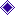Due February 23, 2007Chapter 2 exercises are on pages 87 through 105.

## Live Links to the ExercisesExercise 2.20Exercise 2.23Exercise 2.26Exercise 2.57Exercise 2.69Exercise 2.83Exercise 2.97

## Exercise 2.20

The non-inverting op-amp of figure P2.20 on page 88 shows a DC reference source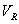in series with the resistor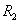, which has five times the resistance of the resistor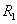.  We use Ohm’s law to find the current through, the reference source, andas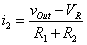.

Using the perfect op-amp principle of the virtual short, we know that this current has a voltage drop throughthat is equal to the input voltage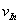.  Setting these equal, we find thatwhich tells us that the effect of the DC reference source as placed in the circuit shown in Figure P2.20(a) is to add that voltage to the output.

We now examine the circuit of Figure P2.20(b).  Placing the source in the branch withinstead produces the same current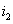but the voltage at the non-inverting input is the drop acrossplus the voltage.  From the virtual short principle, we have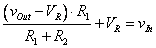.

Solving this equation for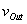we haveWe see that the DC source has more effect on the output in the configuration shown in Figure P2.20(b).

## Exercise 2.23

We could use the results of problem 2.20(b) here but we will instead follow the step-by-step instructions in the problem statement, using the previous homework problem as background and illumination.

We have the non-inverting op-amp of Figure P2.23, in which the voltage divider of a non-inverting op-amp circuit is returned to the input voltage instead of ground.  Part (a) of the problem statement asks for us to find the voltage and current acrossusing the virtual short principle.

Using the virtual short principle, we write the voltages at the inverting and non-inverting inputs to the op-amp as equal,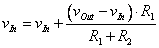from which we immediately see that the current throughis zero, and thus by Ohm’s law the voltage acrossis zero.  The current throughis the same as the current throughand thus is also zero.  Given that the currents throughandare zero, the voltages across them are, by Ohm’s law, zero, and we have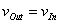.

## Exercise 2.26

We have a circuit, shown in Figure P2.26, in which the output of a non-inverting amplifier is connected to the input through a resistor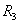and we are asked to find an equation for the current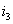through this resistor.  From the equation for the gain of a non-inverting amplifier, we have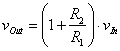.

The currentis, by Ohm’s law,This current flow is back into the battery as a charging current.

## Exercise 2.57

This problem is from the block about Section 2.4.5, Op-Amp Voltage Follower, pages 48-50.  That section uses the perfect op-amp model.  The problem statement is that the op-amp gain K is 200,000, and that the op-amp drives a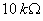load.  Here we use the finite gain equation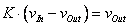.

Note that this equation does not depend on the output load because we are neglecting the output impedance of the op-amp.  From this equation we have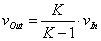so we haveThe current into the load is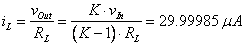.

## Exercise 2.69

We refer to Figure 2.16 page 55, and use the equation for gain as given in Equation (2.49),where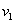andare the input voltages.  Thus the gain is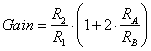.

We are given these values:where c is a constant that may vary from zero to one.  Thus the gain is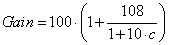.

Thus, the maximum gain for c=0 and the minimum gain for c=1 are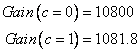which shows a range of gain of almost 10:1.

## Exercise 2.83

We have a hybrid circuit shown in Figure P2.83 page 96, with a two-input non-inverting amplifier added to the circuit of an inverting summing amplifier.  For a circuit withinverting amplifier inputs and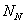amplifier inputs, we can analyze the output by superposition.

For the inverting summation amplifier, we have the circuit treated in detail in Section 2.4.8, Summation Amplifier, on pages 56-59, except that the amplifier has several resistors between the non-inverting input and ground.  Since we neglect the op-amp bias currents with the perfect op-amp model, the inverting summation amplifier is unaffected by the modifications, and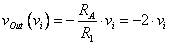for each input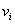.  For the non-inverting amplifier, we have a voltage divider on the input, so the voltage on the non-inverting input isand a non-inverting amplifier with gain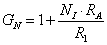so that the total gain is.

If we have another op-amp with an inverting summation for the non-inverting inputs, we can have a circuit that is independent ofbut if we feed it directly to the non-inverting input of our existing amplifier we still have the second gain factor, which is a function of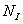.  One simple solution is to have two inverting summation amplifiers, with a difference amplifier as shown in Figure 2.13 page 51 combing their outputs.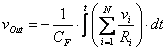.